# Algebra 1 : How to find the common difference in sequences

## Example Questions

### Example Question #1 : How To Find The Common Difference In Sequences

Which of the following cannot be three consecutive terms of an arithmetic sequence?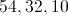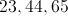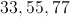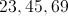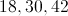Explanation:

In each group of numbers, compare the difference of the second and first terms to that of the third and second terms. The group in which they are unequal is the correct choice.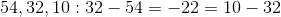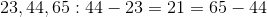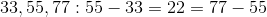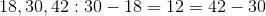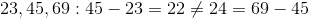The last group of numbers is the correct choice.

### Example Question #2 : How To Find The Common Difference In Sequences

Consider the arithmetic sequence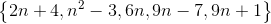If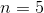, find the common difference between consecutive terms.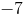Explanation:

In arithmetic sequences, the common difference is simply the value that is added to each term to produce the next term of the sequence. When solving this equation, one approach involves substituting 5 forto find the numbers that make up this sequence. For example,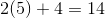so 14 is the first term of the sequence. However, a much easier approach involves only the last two terms,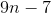and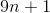.

The difference between these expressions is 8, so this must be the common difference between consecutive terms in the sequence.

### Example Question #3 : How To Find The Common Difference In Sequences

Find the common difference in the following arithmetic sequence.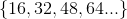Explanation:

An arithmetic sequence adds or subtracts a fixed amount (the common difference) to get the next term in the sequence. If you know you have an arithmetic sequence, subtract the first term from the second term to find the common difference.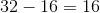### Example Question #4 : How To Find The Common Difference In Sequences

Find the common difference in the following arithmetic sequence.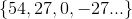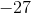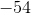Explanation:

An arithmetic sequence adds or subtracts a fixed amount (the common difference) to get the next term in the sequence. If you know you have an arithmetic sequence, subtract the first term from the second term to find the common difference.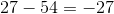(i.e. the sequence advances by subtracting 27)

### Example Question #5 : How To Find The Common Difference In Sequences

What is the common difference in this sequence?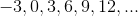Explanation:

The common difference is the distance between each number in the sequence. Notice that each number is 3 away from the previous number.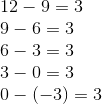### Example Question #6 : How To Find The Common Difference In Sequences

What is the common difference in the following sequence?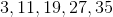Explanation:

What is the common difference in the following sequence?Common differences are associated with arithematic sequences.

A common difference is the difference between consecutive numbers in an arithematic sequence. To find it, simply subtract the first term from the second term, or the second from the third, or so on...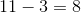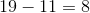See how each time we are adding 8 to get to the next term? This means our common difference is 8.

### Example Question #7 : How To Find The Common Difference In Sequences

What is the common difference in the following sequence: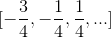Explanation:

The common difference in this set is the linear amount spaced between each number in the set.

Subtract the first number from the second number.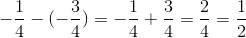Check this number by subtracting the second number from the third number.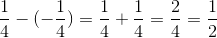Each spacing, or common difference is:### Example Question #8 : How To Find The Common Difference In Sequences

What is the common difference?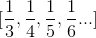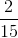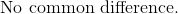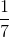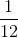Explanation:

The common difference can be determined by subtracting the first term with the second term, second term with the third term, and so forth.   The common difference must be similar between each term.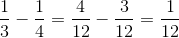The distance between the first and second term is.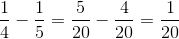The distance between the second and third term is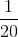.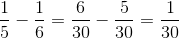The distance between the third and fourth term is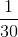.

The fractions may seem as though they have a common difference since the denominators are increasing by one for each term, but there is no common difference among the numbers.

The answer is:### Example Question #9 : How To Find The Common Difference In Sequences

What is the common difference in the following set of data?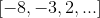Explanation:

In order to determine the common difference, subtract the first term from the second term.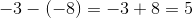Verify that this is the same for the difference of the third and second terms.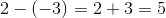The set of data is increasing at increments of five.

The common difference is: